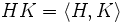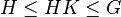# Maximal permutable implies normal

This article gives the proof of a maximality equivalence. In other words, there are two subgroup properties: a stronger one (Normal subgroup (?)) and a weaker one (Permutable subgroup (?)). However, any subgroup maximal among the proper subgroups with the weaker property also has the stronger property, and is thus also maximal among proper subgroups with the stronger property.
View other such statements

## Statement

Suppose$G$ is a group and$H$ is maximal among the proper Permutable subgroup (?)s of$G$. Then,$H$ is a normal subgroup of$G$.

## Proof

Given: A group$G$, a subgroup$H$ that is maximal among the proper permutable subgroups of$G$.

To prove:$H$ is normal in$G$: Any conjugate$K$ of$H$ in$G$ is contained in$H$.

Proof:

1.$K$ is also permutable: This is because conjugation is an automorphism, so conjugate subgroups share the same properties.
2.$HK = \langle H, K \rangle$ is also permutable: This follows from fact (1).
3.$HK \ne G$: This follows from fact (2).
4.$HK = H$: Since$H \le HK \le G$ and$HK$ is permutable, maximality of$H$ forces$HK = H$ or$HK = G$. The latter case is ruled out by the previous step, so$HK = H$.
5.$K \le H$: This follows from the previous step.